# DC Circuit

Direct current (DC) and alternating current (AC) are the two main types of electricity. DC circuit and AC circuit show the structure of the respective circuit systems. Elements of a DC circuit are mainly resistive, which contains a DC battery, a switch, ammeter, voltmeter, a load lamp and connecting leads.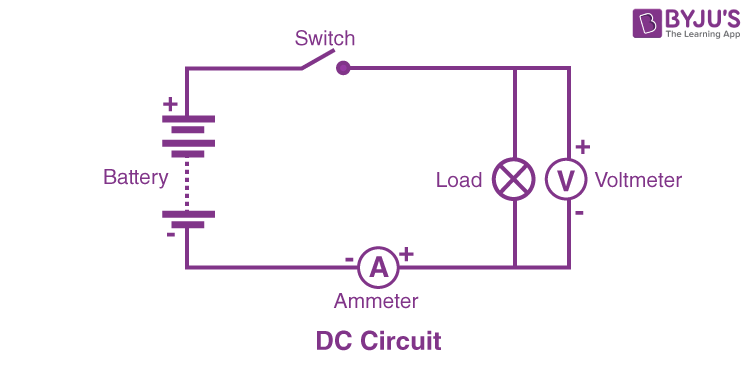## DC Current

Direct current refers to the unidirectional propagation of electric charge. It is commonly used in batteries and solar cells. Thomas Edison invented DC current, which allowed him to power numerous complex electrical systems.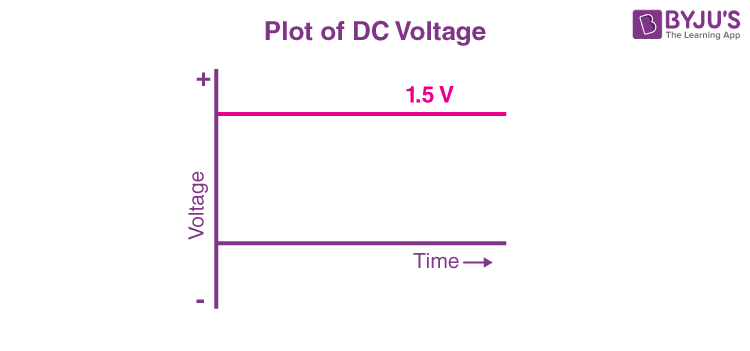## DC Circuit Types

Every electrical circuit can be classified into three groups – series, series-parallel and parallel. Thus, DC circuits can be divided into three categories: series DC circuit, series and parallel DC circuit, and parallel DC circuit.

## Series DC Circuit

When components are connected end to end, they are called a series circuit. In series DC circuits, resistive elements are connected in an end to end way, creating a linear path for flowing current.

Here, resistors

$$\begin{array}{l}R_{1}\end{array}$$
,
$$\begin{array}{l}R_{2}\end{array}$$
and
$$\begin{array}{l}R_{3}\end{array}$$
are attached in series over a voltage source of V volts. Electrical current ‘I’ is running through the given resistors.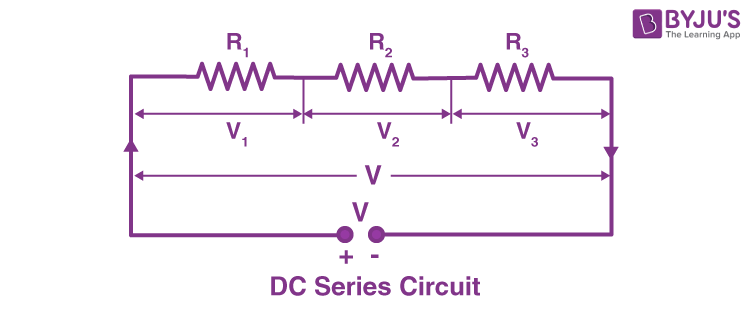Consider

$$\begin{array}{l}V_{1}\end{array}$$
,
$$\begin{array}{l}V_{2}\end{array}$$
and
$$\begin{array}{l}V_{3}\end{array}$$
as the voltage drops over the resistors
$$\begin{array}{l}R_{1}\end{array}$$
,
$$\begin{array}{l}R_{2}\end{array}$$
and
$$\begin{array}{l}R_{3}\end{array}$$
.

Then

$$\begin{array}{l}V = V_{1} + V_{2} + V_{3}\end{array}$$

$$\begin{array}{l}V = IR_{1} + IR_{2} + IR_{3}\end{array}$$

If R is the total circuit resistance, then

$$\begin{array}{l}IR = IR_{1} + IR_{2} + IR_{3}\end{array}$$

$$\begin{array}{l}R = R_{1} + R_{2} + R_{3} \end{array}$$

The total effective resistance is equal to the sum of all individual resistances.

In these types of circuits, the entire system is controlled by a single switch. We cannot individually control each section of the circuit.

• When numerous electrical elements are attached in series, current propagates through all the circuit elements.
• Effective voltage over a series DC circuit is always directly proportional to its effective resistance value.
• The applied voltage over a series circuit is equivalent to the aggregate total of voltage drops over each element.

## Parallel DC Circuit

When multiple electrical components are attached in parallel, one end of each element is attached to a common point, and the other end is attached to a different common point. Here one end of all resistors are connected to a common point, and the remaining ends are connected to another common point (current flows through them).

All the components will have an equal voltage drop over them, and it will be equivalent to the voltage between the two common joints where the components are joined.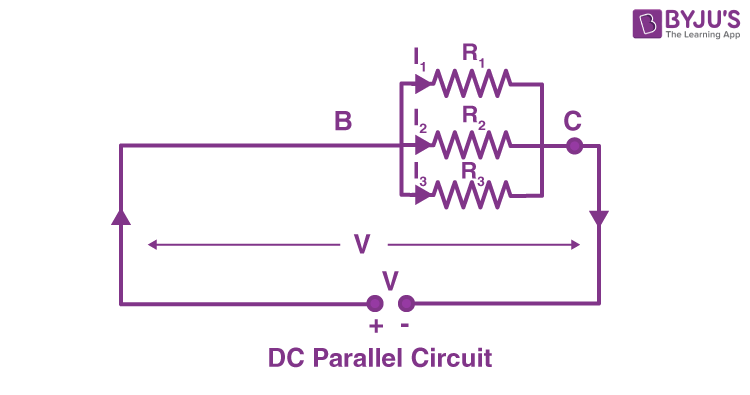In the above circuit diagram, resistors R1, R2 and R3 are joined in parallel over a V voltage supply. I1, I2, and I3 are the currents flowing through them.

The total current flowing through the circuit is,

$$\begin{array}{l}I = I_{1} + I_{2} + I_{3}\end{array}$$

$$\begin{array}{l}I = \frac{V}{R_{1}} + \frac{V}{R_{2}} +\frac{V}{R_{3}} \end{array}$$

Then total electrical resistance R,

$$\begin{array}{l}\frac{V}{R} = \frac{V}{R_{1}} + \frac{V}{R_{2}} +\frac{V}{R_{3}} \end{array}$$

$$\begin{array}{l}\frac{1}{R} = \frac{1}{R_{1}} + \frac{1}{R_{2}} + \frac{1}{R_{3}} \end{array}$$

• Voltage drops are equal over all the components attached in parallel.
• Current through separate components attached in parallel is inversely proportional to their resistances.
• The total effective circuit current is the total sum of the currents flowing through individual elements attached in parallel.
• The reciprocal of total resistance is equal to the aggregate of the reciprocals of the resistances of individual electrical elements attached in parallel.

## Series-Parallel DC Circuit

In reality, electrical circuits are usually a combination of both series and parallel DC circuits. These complex circuits are solved using Ohm’s Law and rules for parallel and series DC circuits.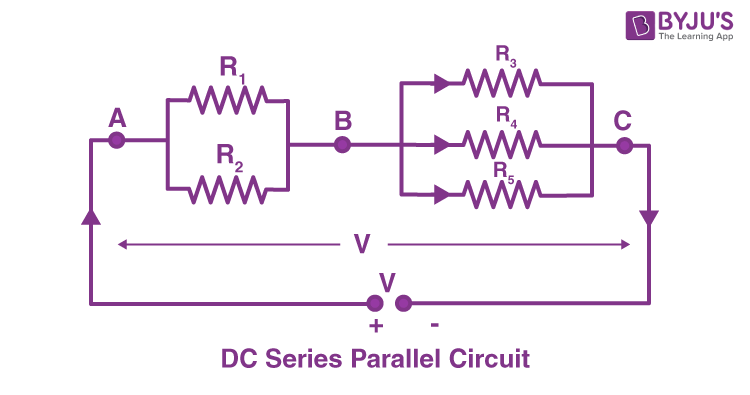In the above circuit diagram, resistors R1 and R2 are joined parallel over the terminal AB. Resistor R3, R4, and R6 are attached in parallel with each other over the terminal BC.

The two batches of resistors RAB and RBC are joined in series with each other over the source voltage V. The effective total resistance of the entire circuit can be calculated as given below,

$$\begin{array}{l}\frac{1}{R_{AB}} = \frac{1}{R_{1}} + \frac{1}{R_{2}} = \frac{R_{1}+R_{2}}{R_{1}R_{2}} \end{array}$$

$$\begin{array}{l}R_{AB} = \frac{R_{1}R_{2}}{R_{1}} + R_{2}\end{array}$$

$$\begin{array}{l}\frac{1}{R_{BC}} = \frac{1}{R_{3}} + \frac{1}{R_{4}} + \frac{1}{R_{5}} = \frac{R_{3}R_{4} + R_{4}R_{5} + R_{5}R_{3}} {R_{3}R_{4}R_{5}}\end{array}$$

$$\begin{array}{l}R_{BC} = \frac{R_{3}R{4}R_{5}} {R_{3}R_{4} + R_{4}R_{5} + R_{5}R_{3}}\end{array}$$

The effective total resistance of the circuit

$$\begin{array}{l}R = R_{AB} + R_{BC}\end{array}$$

Video about the various factors affecting resistance## Frequently Asked Questions on DC Circuit

### What are the main elements or components of a DC circuit?

Elements of a DC circuit are mainly resistive, which contains a DC battery, a switch, ammeter, voltmeter, a load lamp and connecting leads.

### How many switches are in a series DC circuit?

There is only one switch in a series DC circuit. A single switch controls the entire circuit. We cannot individually control each section of the circuit.

### What are the three types of DC circuits?

DC circuits can be divided into three categories: series DC circuit, series and parallel circuit, and parallel DC circuit.

### What is a resistor?

It is an electrical unit with two ends that are operated for either limiting or controlling the flow of current in electrical circuits.

### What is a voltage?

In simple terms, it is the energy that pushes electric current into a conducting coil from the power source of an electrical circuit.

Stay tuned to BYJU’S and Fall in Love with Learning!